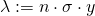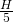# Helmholtz-Lagrange Invariant

is a mapping invariant of paraxial optics, given by the productwhere n is the refraction index,is the aperture angle, and y is the object height.is also called “etendue

This value doesn’t change, if the object side values are replaced by the corresponding image side values:From this we get the paraxial Magnification:# hyperfocal distance

When a lens is focussed to the hyperfocal distance H, the DOF of the lens is maximized: The range of acceptable sharpness then extends fromto infinity

There are two Formulas in use:For f=50mm, F2, and CoC = 0.03mm we getThis is the formula we use here. The results just differ in the focal length of the lens.

andFor f=50mm, F2, and CoC = 0.03mm we getWhere CoC is the circle of confusion, F is the F-number and f is the focal length of the lens.

The hyperfocal distance has curious mathematical properties:

The hyperfocal distance H is the distance at which you have to focus an object to receive the largest depth of field. If, namely, a lens is focussed to H, it is focused fromto infinity.

When focussed to, so everything fromtofocused.
When focussed to, so everything fromtofocused.
When focussed to, so everything fromtofocused.

When focussed to, so everything fromtofocused.

The distanceis the Depth of field.

Notice:

The depth of field is getting smaller, the larger is n, say the shorter the working distance is!